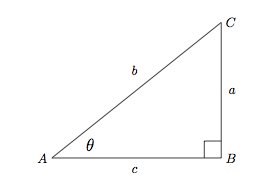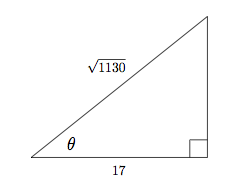# Inverse Trigonometric Functions

## Definition

Inverse Trigonometric Functions are, like any other inverse function, mathematical operators that undo another function's operation.

Given a triangle like thisTriangle ABC

the basic trigonometric functions would be defined as:

$\begin{array}{lcr} \sin \theta = \frac{a}{b} & \cos \theta = \frac{c}{b} & \tan \theta = \frac{a}{c} \end{array}$

with the angle as their input (or argument) and a ratio of sides as their result. However, the inverse functions take the ratio as input and return the angle:

$\sin^{-1} \left( \frac{a}{b} \right) = \theta \\ \cos^{-1} \left( \frac{c}{b} \right) = \theta \\ \tan^{-1} \left( \frac{a}{c} \right) = \theta$

This means the inverse trigonometric functions are useful whenever we know the sides of a triangle and want to find its angles.

Note: The notation $\sin^{-1}$ might be confusing, as we normally use a negative exponent to indicate the reciprocal. However, in this case, $\sin^{-1} \alpha \neq \frac{1}{\sin \alpha}$. When we want the reciprocal of $\sin$ we use $\csc$. In order to avoid this ambiguity, sometimes people might choose to write the inverse functions with an arc prefix. For example:

$\arccos \beta = \cos^{-1} \beta$

## Technique

### In following statement, $a$ and $b$ are positive, co-prime integers. What is the sum of $a$ and $b$?

$\cos^{-1} \frac{17}{\sqrt{1130}}= \tan^{-1} \frac{a}{b}$

Since we are trying to find $a$ and $b$, we should take the tangent of both sides of the equation:

\begin{aligned} \tan \left( \cos^{-1} \frac{17}{\sqrt{1130}} \right) &= \tan \left( \tan^{-1} \frac{a}{b} \right) \\ \tan \left( \underbrace{\cos^{-1} \frac{17}{\sqrt{1130}}}_{\large{\theta}} \right) &= \frac{a}{b} \end{aligned}

Further, we we can use the ratio given to sketch the triangle with $\theta$ in it, using the definition of $\cos^{-1}$:Right Triangle with side 17 and hypotenuse square root 1130

Now, using the Pythagorean theorem, we can see that $17^2 + a^2 = 1130$. This means $a=\sqrt{1130-289}=29$. Finally, we evaluate $\tan \theta = \frac{29}{17}$, which means $a+b=46$. $_\square$Note by Arron Kau
7 years, 4 months ago

This discussion board is a place to discuss our Daily Challenges and the math and science related to those challenges. Explanations are more than just a solution — they should explain the steps and thinking strategies that you used to obtain the solution. Comments should further the discussion of math and science.

When posting on Brilliant:

• Use the emojis to react to an explanation, whether you're congratulating a job well done , or just really confused .
• Ask specific questions about the challenge or the steps in somebody's explanation. Well-posed questions can add a lot to the discussion, but posting "I don't understand!" doesn't help anyone.
• Try to contribute something new to the discussion, whether it is an extension, generalization or other idea related to the challenge.

MarkdownAppears as
*italics* or _italics_ italics
**bold** or __bold__ bold
- bulleted- list
• bulleted
• list
1. numbered2. list
1. numbered
2. list
Note: you must add a full line of space before and after lists for them to show up correctly
paragraph 1paragraph 2

paragraph 1

paragraph 2

[example link](https://brilliant.org)example link
> This is a quote
This is a quote
    # I indented these lines
# 4 spaces, and now they show
# up as a code block.

print "hello world"
# I indented these lines
# 4 spaces, and now they show
# up as a code block.

print "hello world"
MathAppears as
Remember to wrap math in $$ ... $$ or $ ... $ to ensure proper formatting.
2 \times 3 $2 \times 3$
2^{34} $2^{34}$
a_{i-1} $a_{i-1}$
\frac{2}{3} $\frac{2}{3}$
\sqrt{2} $\sqrt{2}$
\sum_{i=1}^3 $\sum_{i=1}^3$
\sin \theta $\sin \theta$
\boxed{123} $\boxed{123}$

Sort by:

Can you please give me inverse formulas of sine cosine tan

- 7 years, 3 months ago